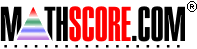Math Practice Online > free > lessons > California > 4th grade > Multiplication Facts Strategies

Here are some tips for Multiplication Facts Strategies, which aligns with California state standards:

Multiplication Facts Strategies

Multiply by 2
Double the number. Any number multiplied by 2 becomes an even number.
3 × 2   =   3 + 3   =    6
5 × 2   =   5 + 5   =   10
8 × 2   =   8 + 8   =   16
Multiply by 5
Use the minute hand on a clock or skip count by 5. Any number multiplied by 5 only ends in "0" or "5".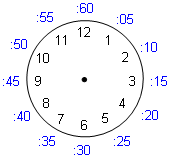3 × 5     When the minute hand is on 3, it is 15 minutes after the hour.     3 × 5 = 15
5 × 5     When the minute hand is on 5, it is 25 minutes after the hour.     5 × 5 = 25
8 × 5     When the minute hand is on 8, it is 40 minutes after the hour.     8 × 5 = 40
 Skip count For 3 × 5, 5, 10, 15, ... 3 × 5 = 15 For 5 × 5, 5, 10, 15, 20, 25, ... 5 × 5 = 25 For 8 × 5, 5, 10, 15, 20, 25, 30, 35, 40, ... 8 × 5 = 40
Odd numbers multiplied by 5 end in "5" and even numbers multiplied by 5 end in "0".

Multiply by 10
Add a zero to the end of the number.

3 × 10 = 30
8 × 10 = 80
123 × 10 = 1230
Multiply by 9
Use your hands by putting both hands out in front of you.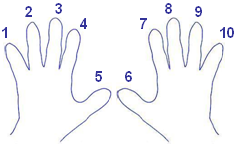Bend the finger of the number you want to multiply. Then count the number of fingers on both sides of the bent finger to get the answer.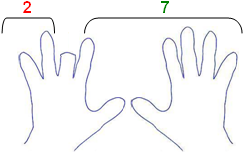For 3 × 9, bend Finger 3. 3 × 9 = 27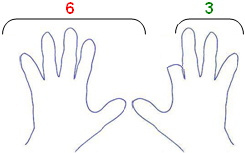For 7 × 9, bend Finger 7. 7 × 9 = 63
Note: For multiples of 9, the digits add up to 9.
 3 × 9 = 27  →   2 + 7 = 9 7 × 9 = 63  →   6 + 3 = 9

Multiply by 4
Double the number twice.
 Doubling once Doubling twice For 3 × 4, 3 × 2 = 6or3 + 3 = 6 → 6 × 2 = 12or6 + 6 = 12 3 × 4 = 12 For 5 × 4, 5 × 2 = 10or5 + 5 = 10 → 10 × 2 = 20or10 + 10 = 20 5 × 4 = 20 For 8 × 4, 8 × 2 = 16or8 + 8 = 16 → 16 × 2 = 32or16 + 16 = 32 8 × 4 = 32
Multiply by 3
Skip count by 3.
 Skip count For 3 × 3, 3, 6, 9, ... 3 × 3 = 9 For 5 × 3, 3, 6, 9, 12, 15, ... 5 × 3 = 15 For 8 × 3, 3, 6, 9, 12, 15, 18, 21, 24, ... 8 × 3 = 24
Multiply by 6